How to use fx-CG50 to back up algebraic work done on paper - Casio Calculators

# How to use fx-CG50 to back up algebraic work done on paper

Mar 2023 Longer Read: 9 MinPeople tend to double-check what they do, let it be in their daily activities or during a Maths exam. For example, it’s natural for students to ask themselves questions such as “Did I put the correct sign for the answer?” and “Did I substitute the correct value for the calculation?” While these seem questions of paranoia, students may make small mistakes halfway through a question, which cost them big marks in their final answer. That’s why the fx-CG50 calculator has many features to back up and verify the algebraic work done on pen and paper, to avoid errors and save time.

In this article, we’ll discuss some ways, as suggested by our Maths Expert Simon May, that students can use to back up their algebraic work to check and verify the calculated values on paper against those calculated using the handset.

## SolveN – on paper and handset!

The powerful “SolveN” function can solve equations numerically for a given variable and, therefore, can be used to make sure the calculations done on paper by the students are exactly correct by checking whether they get the same solutions as the handset.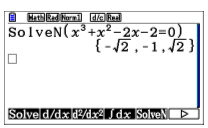## Roots of a function

Students can easily get a function’s roots, either numerically (using SolveN) or graphically, using the graphing menu. They can then check whether the obtained values are the same as the ones they got on paper. To visually confirm the number of roots and further verify what they have got by calculating on paper and numerically using the handset, they can use the graphing feature in the handset.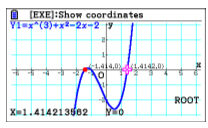Students can easily copy and paste the required mathematical function on the graphing menu or manually enter it, and plot it.

Since not all functions are alike, the viewing V-window can be adjusted to get the function on the screen as needed. By using the `Shift` and then `F3` buttons, students can make various adjustments such as changing the scale of the x and y axes, moving the graph left or right, up or down and adjusting the size of the viewing window. This feature is very useful for better viewing a graphed function.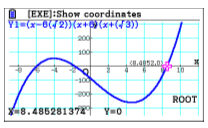To obtain various parameters related to a function, such as the minimum, maximum and roots of the function etc., students can use the `Shift` and then the `G-Solv` (F5) button. In this menu, in addition to visually seeing the roots of the function on the screen, students can use the keypad arrows to jump between the roots to see their numerical values as well. This gives students assurance of their answers obtained on paper and backs them up.

Simon explains, “This would be very important for students because, in Math exams, it’s quite common that they have to use answers from previous calculations in further steps of the question.” Therefore, by verifying the values obtained so far in a question, students can make sure the answers they have obtained are correct and fix any erroneous answers if present, without having to realise that much later in a question, which would be too late in an exam.

## Tangents don’t have to stop you

Asking to find the gradient of a curve at a certain point is also a very common type of question in Math exams. Another similar question is, “What is the equation of the tangent line at point x?”

With the fx-CG50, finding answers to these questions is very easy once Derivative is turned on in the settings. This is thanks to the ability to sketch a tangent line at any point in the function using the handset. Students can access this feature by pressing `Shift` and then `Sketch` (F4) and selecting `Tangent` (F2). After entering the tangent mode, moving the tangent line along the function is very easy using the keypad arrows. The on-screen cursor should help students in the manoeuvre get a reference point. It’s also possible to place the cursor (and thereby the tangent) at a specific point on the function by simply entering the value.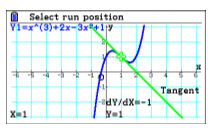It’s sometimes possible to obscure the values (for example, the gradient value) on the screen by the tangent line. This can be easily avoided simply by adjusting the view window or panning the graph using the keypad arrows.

An interesting pro tip from Simon: “Students definitely don’t want to have these values obscured, especially the gradient value, because they can use this value to check what they have obtained by differentiating the initial function. This is really interesting because the answer obtained by differentiating the function should be equal to the gradient value of the tangent line.”

As mentioned earlier, the examiners can take the question a step further by asking the equation of this tangent line. To get the answer to this seemingly-difficult question, all that the students have to do is place the tangent line so that it touches the function at a point of interest, and just press the `EXE` button. The fx-CG50 will solve the problem and give the equation of the tangent line on the screen within seconds. Students can use this feature to back up their calculations.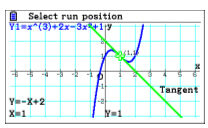### Tables for dynamic values

Simon’s favourite mode on the fx-CG50 is the ‘Table’ function, he says.

Tables can be really important for students when approaching problems. In the Table menu, students can set some configuration values related to the table, such as start, end and step. Usually, the table consists of two columns by default, one for X and one for Y values. If you turn the Derivatives on, an additional column will contain the derivative values. By interpreting the values in the table, students can back up and confirm the calculated values that they have got.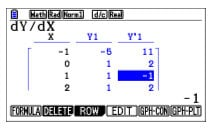The interesting thing about tables is that the students can see a lot of different combinations of input and output values related to a function and, thus no need to check them one by one, which would save a lot of time for them during an exam, and also reduce room for errors.

Another common question is finding the “y” value for a particular “x” value. By using the table, students have to just update the relevant “x” value, and the other values are updated automatically almost instantly.

### Some tips to keep in mind

It’s common for students to have to solve somewhat complex formulae in an exam, such as when applying the trapezium rule. Therefore, they should be extra careful when verifying the answers obtained on paper. Sometimes there can be a mismatch between what students have and the calculated values using a handset, just because of an error in the order of operations.

Simon recommends using brackets to group expressions and perhaps starting from the right side of a formula to check the answers part-way, to avoid such errors. This would benefit students because they may have to solve each part of a complex formula separately to get marks for each part in an exam anyways.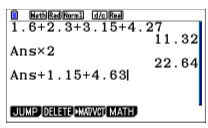Also, the `Shift` and then the `Ans` (-) buttons can be used to use the answer from the immediate earlier step.

### Scary differentiation? fx-CG50 has got you covered

Students can go to the differentiation menu using `OPTN`, then `CALC` (F4) and then the differentiation (F2) button. This differentiation solver option is very helpful in answering “What is the value of the function “Y1 when x equals some value”-type questions.

Here, students can simply enter the function to be differentiated. What’s more interesting is there are several ways of entering the function. They can either directly enter the function or use the variable related to a particular function (say Y1, for example) if already defined in the graph mode. Using a variable could save time and avoid errors because entering a function directly by hand is always a hassle, and why not reuse it if it’s already defined in graph mode?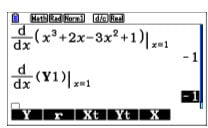If the examiners feel like testing the students with advanced questions, they might ask to differentiate an earlier function after transforming it. With fx-CG50, this problem could easily be approached by going to the graph mode and assigning the transform function to a new variable (say Y2). For instance, if the question asks to transform Y1 to the right by 1 unit, we can write the new function as Y2 = Y1(x-1), as in the image below.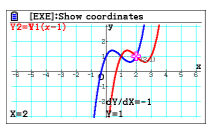### Magic powers

The features mentioned above are like magic powers. Not everyone knows them, and the students who do should be more confident that their understanding and calculations are accurate

Many students make mistakes when the answer to one part of a question needs to be progressed into a further calculation. fx-CG50 allows to back up and verify the answers obtained by students in a question frequently required in further calculations. This frees students’ minds from worrying about things such as missing a decimal place or substituting a wrong value in a later question. These are very common, and costly mistakes students make during exams, mostly due to time pressure.

By using the fx-CG50 handset, students can easily get ahead by saving time and improving the accuracy of their answers in an exam with ease!

### Book a training

We brought you these interesting tips and tricks on how to back up and verify students’ on-paper calculations using the fx-CG50 handset so that students will be amazed at what they can achieve quickly. And what’s cooler is that you can be the one to break the good news to them. To quote Simon: “As a teacher, you’re the one to teach your students the shortcuts, and show them how it’s done!”

If you want to help your students achieve even more, why not signup for our free skills training sessions? One good reason you should signup for the training is that experts run these sessions on the calculator, and therefore it will be a great opportunity to learn from them and, yes, for free!